##CS 660: Combinatorial Algorithms Graphs and Network Flows

[To Lecture Notes Index]
San Diego State University -- This page last updated November 16, 1995## Definitions

### Graph

A graph G is a pair (V,E) where
V is a set of vertices (or nodes)
E is a set of edges connecting the vertices
EXAMPLE
V = {1,2,3,4,5}
E = {(1,2), (1,3), (1,4), (1,5), (2,3), (3,4), (4,5),(5,2)}### Digraph

A Digraph is a graph in which each edges has a direction.

EXAMPLE
V = { 1,2,3,4,5}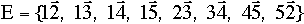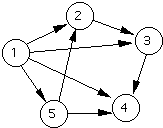### Subgraph

A Subgraph G'= (E',V') of a graph G = (E,V) is a graph where V' is a subset of V and E' is a subset of E### Complete Graph

A graph with an edge between each pair of vertices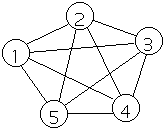```Note for any G = (E,V) we have  0 <= |E| <= |V|*(|V|-1)/2

```

### Path

A path in a graph from vertex V to vertex W is a sequence of edges (V1,V2), (V2,V3),... (Vk-2,Vk-1), (Vk-1,Vk) such that V = V1, and W = Vk. The path is usually given as V1,V2,V3,...Vk
The above path has length k.

### Adjacent Vertices

Two vertices are adjacent if there is an edge connecting them
```
```

### Connected Graph

A graph is connected if there is a path between any two vertices
```
```

### Cycle

A path starting and ending on the same vertex
```
```

### Simple Cycle

A cycle in which all vertices are visited exactly once except the starting vertex: which is visited only twice
```
```

### Weighted Graph

A weighted graph G = (V,E,W) is a graph where each edge e in E has a weight denoted by W(e)

##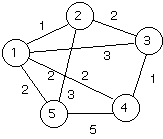The weight of a Graph is the sum of the weights of the edges of the graph

## Representation of Graphs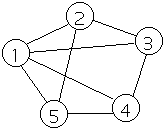```
```

### List of edges

EXAMPLE
1, 2
1, 3
1, 4
1, 5
2, 3
3, 4
4, 5
5, 2

Space - O(|E|) ( actually 2|E|)

### Adjacency Matrix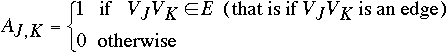1 2 3 4 5 1 0 1 1 1 1 2 1 0 1 0 1 3 1 1 0 1 0 4 1 0 1 0 1 5 1 1 0 1 0

Space - O(|V|2) (can be ~ |V|2/2)

For weighted graph### Adjacency list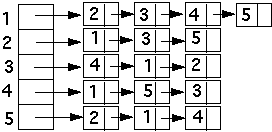Space - O(|E| + |V|)

### Incidence Matrix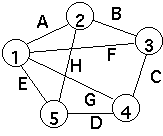A B C D E F G H 1 1 0 0 0 1 1 1 0 2 1 1 0 0 0 0 0 1 3 0 1 1 0 0 1 0 0 4 0 0 1 1 0 0 1 0 5 0 0 0 1 1 0 0 1

Space - O(|E|*|V|)

## Traversing Graphs

### Breath-first(Graph, startNode)

```                                  Line number
for X := 1 to |V| do                      1
Type[X] := unvisited;                  2

Create(Queue);                             3
Type[startNode] := inQueue                 4
Insert(startNode, Queue);                  5
visitOrder := 1;                           6

while not IsEmpty(Queue) do              7
nextNode := GetFirst(Queue);            8
List[nextNode] := visitOrder;           9
visitOrder := visitOrder + 1;          10
Type[nextNode] = visited;              11
for each node adjacent to nextNode do 12
if Type[node] = unvisited then     13
Type[node] := inQueue;           14
Insert(node, Queue);             15
end if;
end for;
end while;

return List
```

## Analysis of Breath-First Search

Measure of size of input: |E| and |V|
Structures used:
```
```
Type
array of size |V|
Each element changed at most three times:
random -> unvisited -> inQueue -> visited

List
array of size |V|
Each element changed once
```
```
Queue
Contains at most |V| - 1 elements
At most |V| adds and |V| deletes from queue
At most |V| calls to IsEmpty
```
```
VisitOrder
Increased |V| times

## Analysis of Breath-First Search - Cont.

```
```
Graph
```Must find all neighbors of each node in graph
```

Adjacency Matrix O(|V|2)
 1 2 3 4 5 1 0 1 1 1 1 2 1 0 1 0 1 3 1 1 0 1 0 4 1 0 1 0 1 5 1 1 0 1 0

Adjacency list O(|E| + |V|)Traversing Graphs

### Depth-first(Graph, startNode)

```                                  Line number
for X := 1 to |V| do                      1
Type[X] := unvisited;                  2

Create(Stack);                             3
Type[startNode] := inStack;                4
Insert(startNode, Stack);                  5
visitOrder := 1;                           6

while not IsEmpty(Stack) do              7
nextNode := Pop(Stack);                 8
List[nextNode] := visitOrder;           9
visitOrder := visitOrder + 1;          10
Type[nextNode] = visited;              11
for each node adjacent to nextNode do 12
if Type[node] = unvisited then     13
Type[node] := inStack;           14
Push(node, Stack);               15
end if;
end for;
end while;
```

## Network Flow

A flow network G = (V, E) is a directed graph where each edge (u, v) [[propersubset]] E has a capacity c(u, v) >= 0Source (s) - node with only outflows

Sink (t) - node with only inflows

Assume graph is connected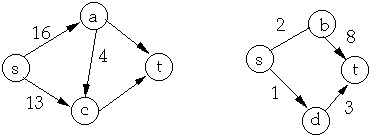Multiple Sources/Sinks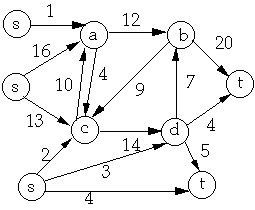Super Sources and Sinks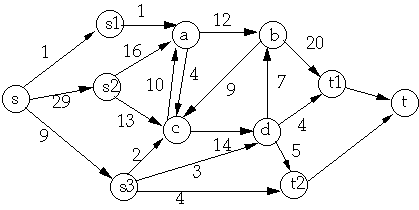A flow in G is a real-valued function f : V * V -> R such that:
• For all u, v [[propersubset]] V, f(u, v) <= c(u, v) [Capacity constraint]
• For all u, v [[propersubset]] V, f(u, v) = -f(v, u) [Skew symmetry]
• For all u [[propersubset]] V - {s, t},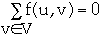[Flow conservation]

Value of a flow f isPositive Net Flow entering vertex v is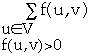Positive Net Flow leaving vertex v isf(a, b) = 9 f(b, a) = -9 c(b, a) = 0 c(a, d) = 0 f(a, d) = 0
Oh Boy More Notation

Implicit Summation Notation

Let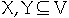then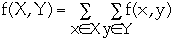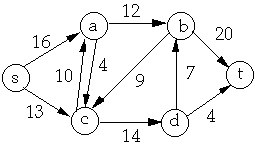X = {a, c}, Y = {b, d } then

f(X, Y) = f(a, b) + f(a, d) + f(c, b) + f(c, b)
= 12 + 0 + -9 + 14
= 17

Lemma. Let G = (V, E) be a flow network and f be a flow in G

a. If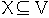then f(X, X) = 0

b. Ifthen f(X, Y) = -f(Y, X)

c. If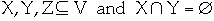thenandproof of a
• For all u [[propersubset]] V f(u, u) = 0 since we have f(u, u) = -f(u, u)
• We have: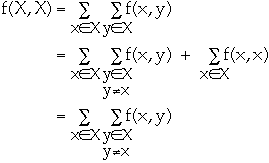For every term f(x, y) we also have f(y, x)
Since f(x, y) = -f(y, x) we have f(X, X) = 0

```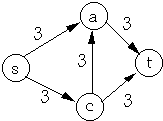```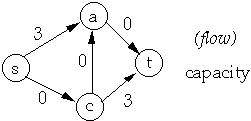Let G = (V, E) be a flow network and f a flow in G

residual capacity of (u, v) is cf(u, v) = c(u, v) - f(u, v)

residual network of G induced by f is Gf = (V, Ef) where
Ef = { (u, v) V * V : cf(u, v) > 0}
Residual Network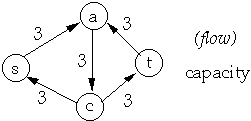Network Flow on Network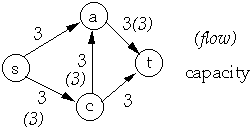Residual Network Flow in Residual Network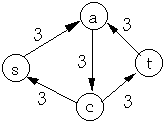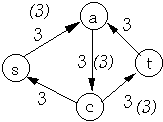Resultant Flow in Original NetworkLet G = (V, E) be a flow network and f be a flow in G.

Let Gf = (V, Ef) be the residual network of G induced by f.

Let h be a flow in Gf.

Define f + h to by (f + h )(u, v) = f(u, v) + h(u, v)

Lemma. f + h is a flow in G with value |f + h|= |f|+|h|

proof of Capacity constraint

(f + h )(u, v) = f(u, v) + h(u, v)

= -f(v, u) - h(v, u)

= -(f(v, u) + h(v, u))

= -(f + h )(v, u)

Augmenting Paths

Let G = (V, E) be a flow network and f be a flow in G.

Let Gf = (V, Ef) be the residual network of G induced by f.

An augmenting path p is a simple path from s to t in Gf

Residual capacity of p is the maximum amount of net flow that we can ship along the edges of p

cf(p) = min { cf(u, v) : (u, v) is on path p}
 Residual Network Flow in Residual NetworkLemma. Let G = (V, E) be a flow network and f be a flow in G.

Let p be an augmenting path in Gf. Define hp : V * V -> R by:Then hp is a flow in Gf with value |hp| = cf(p).

Corollary

Let G = (V, E) be a flow network and f be a flow in G.

Let p be an augmenting path in Gf. Define hp as above.

Let k = f + hp. Then k is a flow in G with value

|k| = |f|+ | hp | > |f|

Ford-Fulkerson Method to find Maximum Flow

Let G = (V, E) be a flow network

initialize flow f to 0

while there exits an augmenting path p
do add hp to f

return f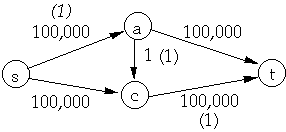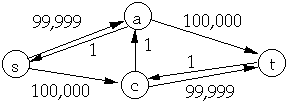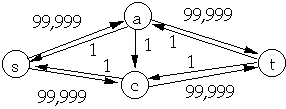Let G = (V, E) be a flow network and f a flow in G

A cut (S, T) of G is a partition of V into S and T = V - S such that:
s [[propersubset]] S and t [[propersubset]] T

The net flow across the cut (S, T) is f(S, T)

The capacity of the cut (S, T) is c(S, T)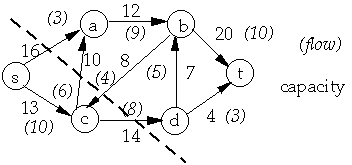S = {s, c} T = { a, b, d, t}

f(S, T) = 3 + 6 - 4 + 8 = 13

c(S, T) = 16 + 10 + 14

Lemma

Let G = (V, E) be a flow network and f be a flow in G.

Let (S, T) be a cut of G. Then the net flow across (S, T) is
f(S, T) = |f|

proof:
f(S, T)
= f(S, V - S)
= f(S, V - S)
= f(S, V) - f(S, S)
= f(S, V)
= f(s, V) + f(S - s, V)
= f(s, V)
= |f|

Corollary The value of any flow f in a flow network G is bounded from above by the capacity of any cut of G

## The Max-flow min-cut theorem

Let f be a flow in G = (V, E) be a flow network with source s and sink t.

The following are equivalent:

1. f is a maximum flow in G

2. The residual network Gf contains no augmenting paths

3. |f| = c(S, T) for some cut (S, T) of G.

Ford-Fulkerson Algorithm to find Maximum Flow

Let G = (V, E) be a flow network

for each edge (u, v) [[propersubset]] E do
f[u, v] = 0
f[v, u] = 0

while there exits a path p from s to t in residual network Gf do
do cf(p) = min { cf(u, v) : (u, v) is on path p}
for each edge (u, v) in p
do
f[u, v] = f[u, v] + cf(p)
f[u, v] = -f[v, u]
return f

How long does it take?

Edmonds-Karp Algorithm
Find shortest path from s to t in Ford-Fulkerson

Let G = (V, E) be a flow network and f be a flow in G.

Let Gf = (V, Ef) be the residual network of G induced by f.

Let p be shortest path from s to t in Gf.

Define the flow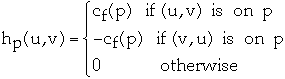Let df(x, y) be the shortest-path distance from x to y in Gf

Let dh(x, y) be the shortest-path distance from x to y in Gh

Lemma 27.8 For all v [[propersubset]] V - {s, t} we have df(s, v) <= dh(s, v)

proof:
Assume that there exists v such that df(s, v) > dh(s, v)
Let v be the closes vertex to s for which this is true
Let sp be the shortest path from s to v in Gf
Let u be the vertex before v on sp and f[u, v] < c(u,v) then
df(s, v)
= df(s, u) + 1
<= dh(s, u) + 1
= dh(s, v)
So we must have f[u, v] = c(u, v)
Thus (u, v) is not in Ef which means (v, u) is in Ef
So df(s, u)
= df(s, v) + 1
df(s, v)
= df(s, u) - 1
<= dh(s, u) - 1
= dh(s, v) - 2
< dh(s, v)

Theorem The Edmonds-Karp Algorithm will perform at most O(V*E) flow augmentations.

proof:
An edge (u, v) in a residual network Gf is critical on an augmenting path p if the residual capacity of p is the residual capacity of (u, v)
How many times can an edge be critical?
We have (draw picture)
df(s, v)
= df(s, u) + 1
dh(s, u)
= dh(s, v) + 1
So dh(s, u)
= dh(s, v) + 1
>= df(s, v) + 1
= df(s, u) + 2
Thus ( u, v) can be critical at most O(V) times
Since there are O(E) edges the total number of critical edges is O(E*V)